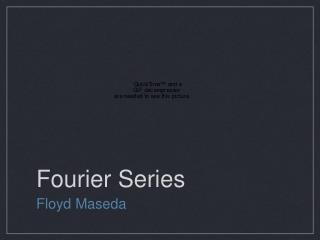DownloadDownload PresentationFourier Series

# Fourier Series

Télécharger la présentation## Fourier Series

- - - - - - - - - - - - - - - - - - - - - - - - - - - E N D - - - - - - - - - - - - - - - - - - - - - - - - - - -
##### Presentation Transcript

1. Fourier Series • Floyd Maseda

2. What is a Fourier Series? • A Fourier series is an approximate representation of any periodic function in terms of simpler functions like sine and cosine. • The series has many applications in electrical engineering, acoustics, optics, processing, quantum mechanics, etc.

3. an and bn are called “Fourier coefficients” and are defined by the following equations: • where L is half the length of the segment being periodically (L=λ/2) repeated and f(x) is a function describing the segment.

4. Example: Triangle Wave • There are two types of triangle waves: Odd waves (such as the one pictured above) and even waves. While both waves are basically the same thing, they differ slightly in what is being repeated. • Even waves repeat a single line while odd waves repeat a “bent” line.

5. EVEN WAVE ODD WAVE

6. The Programs EVEN WAVE ODD WAVE ... DO II=1,100 DO JJ=1,50 COEFF = 2.0*CNUM - 1.0 FAC = (4.0/PI)*COS(COEFF*XX)/(COEFF**2) FUNC = FUNC + FAC CNUM = CNUM + 1.0 END DO WRITE(6,*) XX,FUNC FUNC = 0.0 CNUM = 1.0 XX = XX + 0.1 END DO... ... DO II=1,100 DO JJ=1,50 COEFF = 2.0*CNUM - 1.0 FAC = ((-1)**(CNUM+1.0))*(4.0/PI)*SIN(COEFF*XX)/(COEFF**2) FUNC = FUNC + FAC CNUM = CNUM + 1.0 END DO WRITE(6,*) XX,FUNC FUNC = 0.0 CNUM = 1.0 XX = XX + 0.1 END DO...

7. The Output EVEN WAVE

8. The Output ODD WAVE

9. Another Example: Square Wave • The square wave I attempted to recreate was a representation of an analog-digital conversion of an audio signal.

10. Alterations • In order to make the square wave work, I had to make some alterations to the way I approached the wave. • Since the wave was neither even nor odd, I thought of it as a translation of another function. If the wave was shifted down 1/2 unit, it would work similarly to the previous two waves.

11. The Fourier Series

12. The Output Gibbs Phenomenon: Approximation encounters large oscillations at jump discontinuities in the original function.

13. Nonlinear Fourier Series • To demonstrate the versatility of the Fourier Series, I decided to try a non-linear function. While according to the research I did, anything plugged into the Fourier series that is a function will work, some functions are harder than others to integrate and come up with a sigma representation. • Trying to integrate something with a square root (semicircle, sideways parabola, etc.) is a nightmare even for WolframAlpha!

14. Example: y=x2 The derived Fourier series

15. The Output

16. Summary • Any periodic function can be expressed as a superposition of many simple trigonometric functions • Most of the work involved is actually in integrating the function itself • Some functions are harder to integrate than others